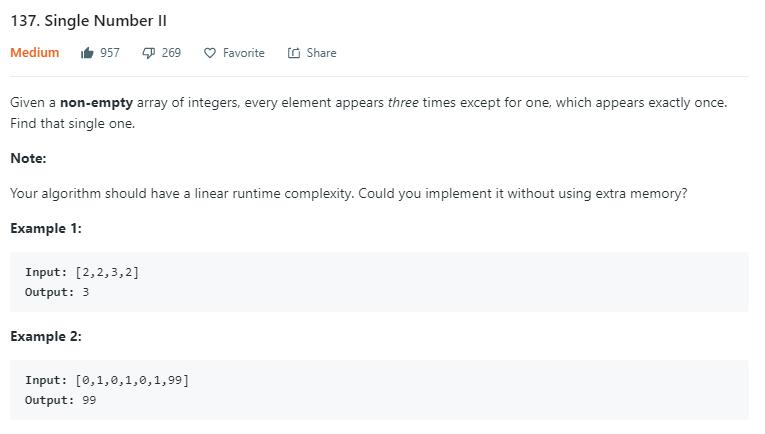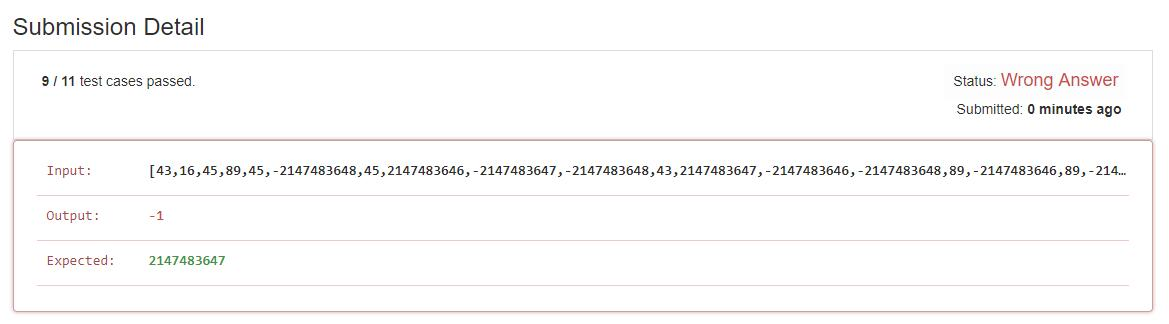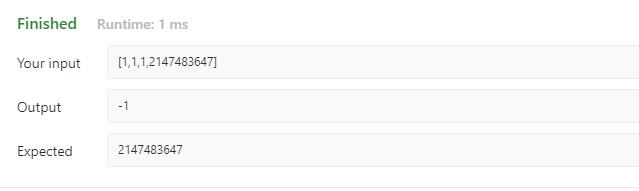# 题目描述（中等难度）136 题 的升级版，这个题的话意思是，每个数字都出现了 3 次，只有一个数字出现了 1 次，找出这个数字。同样要求时间复杂度为 O（n），空间复杂度为 O（1）。

# 解法一

public int singleNumber(int[] nums) {
HashMap<Integer, Integer> map = new HashMap<>();
for (int i = 0; i < nums.length; i++) {
if (map.containsKey(nums[i])) {
map.put(nums[i], map.get(nums[i]) + 1);
} else {
map.put(nums[i], 1);
}
}
for (Integer key : map.keySet()) {
if (map.get(key) == 1) {
return key;
}

}
return -1; // 这句不会执行
}


# 解法二 数学推导

2 * ( a + b + c + d) - (a + b + a + b + c + c + d)，然后结果是不是就是 d 了。。。。。。

public int singleNumber(int[] nums) {
HashSet<Integer> set = new HashSet<>();
int sum = 0;
for (int i = 0; i < nums.length; i++) {
sum += nums[i];
}
int sumMul = 0;
for (int n : set) {
sumMul += n;
}
sumMul = sumMul * 3;
return (sumMul - sum) / 2;
}(1 + Ingeger.MaxValue) * 3 - (1 + 1 + 1 + Integer.MaxValue) = 2 * Integer.MaxValue

2 * Integer.MaxValue = -2，最后我们返回的结果就是 -2 / 2 = -1# 解法三 位操作

136 题通过异或解决了问题，这道题明显不能用异或了，参考 这里-easy-to-understand-solution-easily-extended-to-any-times-of-occurance) 的一个解法。

假如例子是 1 2 6 1 1 2 2 3 3 3, 3 个 1, 3 个 2, 3 个 3,1 个 6
1 0 0 1
2 0 1 0
6 1 1 0
1 0 0 1
1 0 0 1
2 0 1 0
2 0 1 0
3 0 1 1
3 0 1 1
3 0 1 1



public int singleNumber(int[] nums) {
int ans = 0;
//考虑每一位
for (int i = 0; i < 32; i++) {
int count = 0;
//考虑每一个数
for (int j = 0; j < nums.length; j++) {
//当前位是否是 1
if ((nums[j] >>> i & 1) == 1) {
count++;
}
}
//1 的个数是否是 3 的倍数
if (count % 3 != 0) {
ans = ans | 1 << i;
}
}
return ans;
}


# 解法四 通用方法

## 2. 考虑其中的一个 bit

假如例子是 1 2 6 1 1 2 2 3 3 3, 3 个 1, 3 个 2, 3 个 3,1 个 6
1 0 0 1
2 0 1 0
6 1 1 0
1 0 0 1
1 0 0 1
2 0 1 0
2 0 1 0
3 0 1 1
3 0 1 1
3 0 1 1


x1 的变化规律就是遇到 1 变成 1 ，再遇到 1 变回 0。遇到 0 的话就不变。

x2 的话，当遇到 1 的时候，如果之前 x10x2 就不变。如果之前 x11，对应于上边的第二次遇到 1 和第四次遇到 1x20 变成 1 和 从 1 变成 0

x2 = x2 ^ (i & x1)，多判断了 x1 是不是 1

x3，x4 ... xm 就是同理了，xm = xm ^ (xm-1 & ... & x1 & i)

00 -> 01 -> 10 -> 11 -> 00

mask = ~(y1 & y2 & ... & ym),

k = 3: 写成二进制，k1 = 1, k2 = 1, mask = ~(x1 & x2);

k = 5: 写成二进制，k1 = 1, k2 = 0, k3 = 1, mask = ~(x1 & ~x2 & x3);

for (int i : nums) {
xm ^= (xm-1 & ... & x1 & i);
xm-1 ^= (xm-2 & ... & x1 & i);
.....
x1 ^= i;

mask = ~(y1 & y2 & ... & ym) where yj = xj if kj = 1, and yj = ~xj if kj = 0 (j = 1 to m).

......
}


# 考虑全部 bit

假如例子是 1 2 6 1 1 2 2 3 3 3, 3 个 1, 3 个 2, 3 个 3,1 个 6
1 0 0 1
2 0 1 0
6 1 1 0
1 0 0 1
1 0 0 1
2 0 1 0
2 0 1 0
3 0 1 1
3 0 1 1
3 0 1 1


int x1 = 0, x2 = 0, mask = 0;

for (int i : nums) {
x2 ^= x1 & i;
x1 ^= i;
}


## 1. 返回什么

假如例子是 1 2 6 1 1 2 2 3 3 3, 3 个 1, 3 个 2, 3 个 3,1 个 6
1 0 0 1
2 0 1 0
6 1 1 0
1 0 0 1
1 0 0 1
2 0 1 0
2 0 1 0
3 0 1 1
3 0 1 1
3 0 1 1

x1 1 1 0
x2 0 1 1
x3 0 1 1


public int singleNumber(int[] nums) {
int x1 = 0, x2 = 0, mask = 0;
for (int i : nums) {
x2 ^= x1 & i;
x1 ^= i;
}
return x1;
}


public int singleNumber(int[] nums) {
int x1 = 0, x2 = 0, x3  = 0, mask = 0;
for (int i : nums) {
x3 ^= x2 & x1 & i;
x2 ^= x1 & i;
x1 ^= i;
mask = ~(x1 & ~x2 & x3);
}
return x1;
}


136 题 中，k = 2, p = 1 ，其实也是这个类型。只不过因为 k = 2，而我们用一个比特位计数的时候，等于 2 的时候就自动归零了，所以不需要 mask，相对来说就更简单了。

public int singleNumber(int[] nums) {
int x1 = 0;

for (int i : nums) {
x1 ^= i;
}

return x1;
}